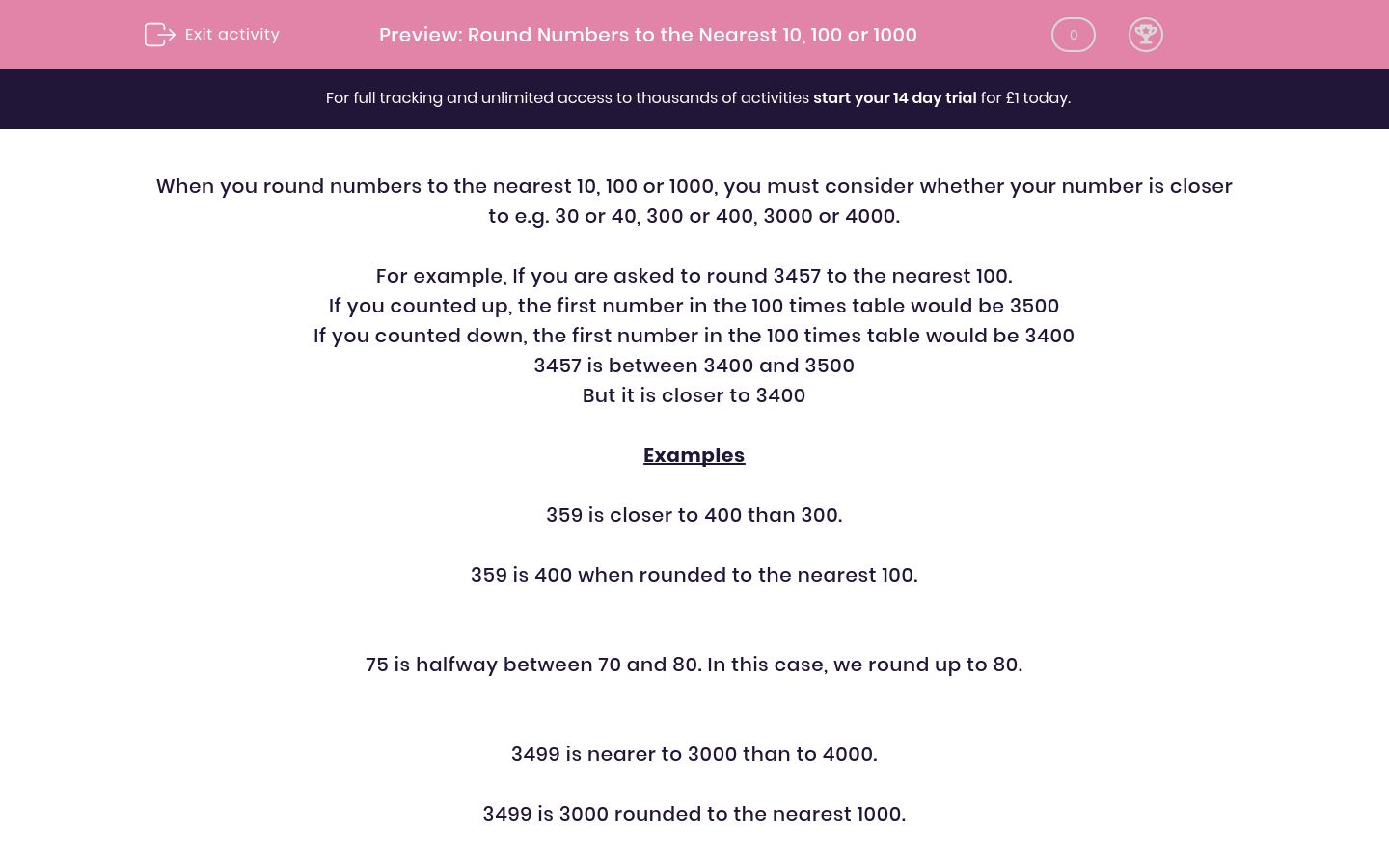# Round Numbers to the Nearest 10, 100 or 1000

In this worksheet, students revise rounding numbers to the nearest 10, 100 or 1000. They should try to work quickly and accurately.Key stage:  KS 2

Curriculum topic:   Maths and Numerical Reasoning

Curriculum subtopic:   Place Value

Difficulty level:### QUESTION 1 of 10

When you round numbers to the nearest 10, 100 or 1000, you must consider whether your number is closer to e.g. 30 or 40, 300 or 400, 3000 or 4000.

For example, If you are asked to round 3457 to the nearest 100.

If you counted up, the first number in the 100 times table would be 3500

If you counted down, the first number in the 100 times table would be 3400

3457 is between 3400 and 3500

But it is closer to 3400

Examples

359 is closer to 400 than 300.

359 is 400 when rounded to the nearest 100.

75 is halfway between 70 and 80. In this case, we round up to 80.

3499 is nearer to 3000 than to 4000.

3499 is 3000 rounded to the nearest 1000.

424 rounded to the nearest 10 is

347 rounded to the nearest 10 is

185 rounded to the nearest 10 is

529 rounded to the nearest 100 is

275 rounded to the nearest 100 is

250 rounded to the nearest 100 is

575 rounded to the nearest 100 is

2178 rounded to the nearest 1000 is

7502 rounded to the nearest 1000 is

506 rounded to the nearest 1000 is

5060 rounded to the nearest 1000 is

5060 rounded to the nearest 100 is

5060 rounded to the nearest 10 is

1499 rounded to the nearest 1000 is

1499 rounded to the nearest 100 is

1499 rounded to the nearest 10 is

4449 rounded to the nearest 1000 is

4449 rounded to the nearest 100 is

4449 rounded to the nearest 10 is

4999 rounded to the nearest 10 is
• Question 1

424 rounded to the nearest 10 is
420
EDDIE SAYS
424 is between 420 and 430 It is closer to 420
• Question 2

347 rounded to the nearest 10 is
350
EDDIE SAYS
347 is between 340 and 350 It is closer to 350
• Question 3

185 rounded to the nearest 10 is
190
EDDIE SAYS
185 is between 180 and 190 As it end in a 5, we always round up. It is closer to 190
• Question 4

529 rounded to the nearest 100 is
500
EDDIE SAYS
529 is between 520 and 530 It is closer to 530
• Question 5

275 rounded to the nearest 100 is
300
EDDIE SAYS
275 is between 200 and 300 It is closer to 300
• Question 6

250 rounded to the nearest 100 is
300
EDDIE SAYS
250 is between 200 and 300 As it exactly half way, we round up.
• Question 7

575 rounded to the nearest 100 is
600
EDDIE SAYS
575 is between 500 and 600 It is closer to 600
• Question 8

2178 rounded to the nearest 1000 is
2000
EDDIE SAYS
2178 is between 2000 and 3000 It is closer to 2000
• Question 9

7502 rounded to the nearest 1000 is
8000
EDDIE SAYS
7502 is between 7000 and 8000 It is, just, closer to 8000
• Question 10

506 rounded to the nearest 1000 is
1000
EDDIE SAYS
506 is between 10 and 1000 It is closer to 1000
• Question 11

5060 rounded to the nearest 1000 is
5000
EDDIE SAYS
5060 is between 5000 and 6000 It is closer to 5000
• Question 12

5060 rounded to the nearest 100 is
5100
EDDIE SAYS
5060 is between 5000 and 5100 It is closer to 5100
• Question 13

5060 rounded to the nearest 10 is
5060
EDDIE SAYS
5060 is already in the 10 times table (it ends in a zero). It is already rounded to the nearest 10.
• Question 14

1499 rounded to the nearest 1000 is
1000
EDDIE SAYS
1499 is between 1000 and 2000 It is closer to 1000
• Question 15

1499 rounded to the nearest 100 is
1500
EDDIE SAYS
1499 is between 1400 and 1500 It is closer to 1500
• Question 16

1499 rounded to the nearest 10 is
1500
EDDIE SAYS
1499 is between 1490 and 1500 It is closer to 1500
• Question 17

4449 rounded to the nearest 1000 is
4000
EDDIE SAYS
4449 is between 4000 and 5000 It is closer to 4000
• Question 18

4449 rounded to the nearest 100 is
4400
EDDIE SAYS
4449 is between 4400 and 4500 It is closer to 4400
• Question 19

4449 rounded to the nearest 10 is
4450
EDDIE SAYS
4449 is between 4440 and 4450 It is closer to 4450
• Question 20

4999 rounded to the nearest 10 is
5000
EDDIE SAYS
4999 is between 4490 and 5000 It is closer to 5000
---- OR ----

Sign up for a £1 trial so you can track and measure your child's progress on this activity.

### What is EdPlace?

We're your National Curriculum aligned online education content provider helping each child succeed in English, maths and science from year 1 to GCSE. With an EdPlace account you’ll be able to track and measure progress, helping each child achieve their best. We build confidence and attainment by personalising each child’s learning at a level that suits them.

Get started